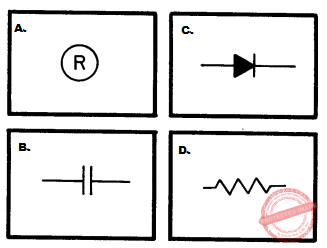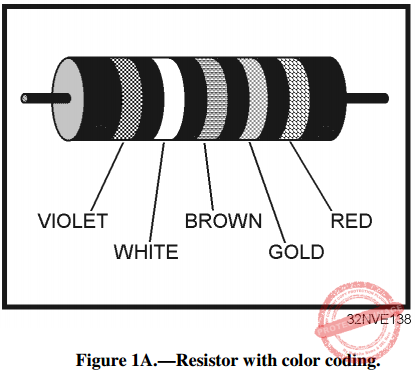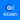# NEETS Module 01 Assignment 1: Turning to Electricity

(Last Updated On: December 8, 2017)

This is the Textbook Assignment: Chapter 1, “Turning to Electricity” from the Module 1 — Introduction to Matter, Energy, and Direct Current in Navy Electricity and Electronics Training Series (NEETS) . If you are looking for a reviewer in Electronics Engineering this will definitely help. I can assure you that this will be a great help in reviewing the book in preparation for your Board Exam. Make sure to familiarize each and every questions to increase the chance of passing the ECE Board Exam.

### Start Practice Exam Test Questions

Choose the letter of the best answer in each questions.

1.  Matter can be found in which of the following forms?

• A.  Solid
• B.  Liquid
• C.  Gaseous
• D.  Each of the above

2.  A substance that CANNOT be reduced to a simpler substance by chemical means is called a/an

• A.  element
• B.  mixture
• C.  compound
• D.  solution

3.  A molecule is the smallest possible particle that retains the characteristic of which of the following substances?

• A.  An element
• B.  A mixture
• C.  A compound
• D.  A solution

4.  An atom is the smallest possible particle that retains the characteristic of which of the following substances?

• A.  An element
• B.  A mixture
• C.  A compound
• D.  A solution

5.  What subatomic particle has a negative charge and a small mass?

• A.  Proton
• B.  Electron
• C.  Positron
• D.  Neutron

6.  What subatomic particle has a positive charge and a large mass?

• A.  Proton
• B.  Electron
• C.  Positron
• D.  Neutron

7.  What subatomic particle has no charge?

• A.  Proton
• B.  Electron
• C.  Positron
• D.  Neutron

8.  When light is represented as a tiny packet of energy, what are these packets of energy called?

• A.  Angstroms
• B.  Photons
• C.  Wavelengths
• D.  Frequencies

9.  If light energy collides with an orbiting electron, what happens to the electron?

• A.  The electron will move around the same orbit faster
• B.  The electron will jump to an orbit further from the nucleus
• C.  The electron will jump to an orbit closer to the nucleus
• D.  The electron will merge with the nucleus

10.  After the action described in question 9 occurs, the electron will return to the condition it had before being acted upon by the light. When the electron returns to this condition, which of the following actions occurs?

• A.  The nucleus becomes lighter
• B.  The atom becomes an ion
• C.  Light energy is emitted
• D.  The valence of the atom changes

11. The number of electrons in the outermost shell of an atom determines which of the following characteristics of the atom?

• A.  Valence
• B.  Atomic weight
• C.  Atomic number
• D.  Number of shells

12. When an atom gains or loses an electron, which of the following terms applies?

• A.  Unbalanced
• B.  Lightened
• C.  Neutral
• D.  Ionized

13. What is the main difference between conductors, semiconductors, and insulators?

• A.  The temperature differences
• B.  The physical state of their mass
• C.  The number of free electrons
• D.  The designations of the outer shells

14.  A substance with an excess of electrons is considered to be in what electrical state?

• A.  Neutral
• B.  Positive
• C.  Negative
• D.  Discharged

15. Which of following actions describes the easiest way to accumulate a static electric charge?

• A.  Friction between two conductors
• B.  Friction between two insulators
• C.  Pressure between two conductors
• D.  Pressure between two insulators

16. An atom that contains 6 protons and 5 electrons has what electrical charge?

• A. Positive
• B. Negative
• C. Neutral
• D. Intermediate

17. How do "like" and "unlike" charges react to one another?

• A. Unlike charges repel each other, like charges repel each other
• B. Unlike charges attract each other, like charges attract each other
• C. Unlike charges repel each other, like charges attract each other
• D. Unlike charges attract each other, like charges repel each other

18. What is/are the term(s) applied to the space between and around charged bodies in which their influence is felt?

• A. Electric field of force
• B. Electrostatic field
• C. Dielectric field
• D. Each of the above

19. Electrostatic lines of force are drawn in which of the following manners?

• A. Entering negative charge, entering positive charge
• B. Entering negative charge, leaving positive charge
• C. Leaving negative charge, leaving positive charge
• D. Leaving negative charge, entering positive charge

20. Which of the following devices use magnetism?

• A. Batteries
• B. Light bulbs
• C. High-fidelity speakers
• D. Each of the above

21. Magnetic materials have which of the following qualities?

• A. They are attracted by magnets
• B. They can be magnetized
• C. Both A and B above
• D. They are electrical insulators

22. Ferromagnetic materials have which of the following qualities?

• A. They are all alloys
• B. They all contain nickel
• C. They make very weak magnets
• D. They are relatively easy to magnetize

23. A material with low reluctance and high permeability such as iron or soft steel is used to make what type of magnet?

• A. Temporary
• B. Permanent
• C. Residual
• D. Natural

24. The ability of a material to retain magnetism is called

• A. permeability
• B. retentivity
• C. reluctance
• D. ionization

25. The law of magnetic poles states which of the following relationships?

• A. Like poles attract, unlike poles attract
• B. Like poles attract, unlike poles repel
• C. Like poles repel, unlike poles repel
• D. Like poles repel, unlike poles attract

26. The north indicating pole of a compass needle is attracted to which of the following poles of the earth?

• A. The geographic north pole
• B. The magnetic north pole
• C. The geographic south pole
• D. The magnetic south pole

27. Weber’s theory of magnetism assumes that magnetic material is composed of

• A. tiny molecular magnets
• B. domains of magnetic influence
• C. large blocks of material acting as magnets
• D. atoms with electrons spinning different directions

28. According to the domain theory, if an atom with 26 electrons has 20 electrons spinning counterclock-wise, the atom is considered to be

• A. charged
• B. insulated
• C. neutralized
• D. magnetized

29. If a glass plate is placed over a magnet and iron filings are sprinkled over the glass, a pattern will be visible. What does this pattern indicate?

• A. The magnetic field
• B. The electrostatic field
• C. The piezoelectric effect
• D. The chemical reaction of the magnet and the filings

30. An imaginary line used to illustrate a magnetic effect is known as a/an

• A. magnetic pole
• B. force field pole
• C. magnetic line of force
• D. electrostatic line of force

31. Which of the following is NOT a property of magnetic lines of force?

• A. They form closed loops around the magnet
• B. They leave the magnetic material at right angles to the surface
• C. They cross each other at right angles
• D. They leave the north pole and enter the south pole of the magnet

32. A magnetic shield or screen used to protect a delicate instrument should be made of which of the following materials?

• A. Plastic
• B. Copper
• C. Soft iron
• D. Aluminum

33. Bar magnets should be stored in which of the following manners?

• A. Separately
• B. In pairs at 90 degree angles
• C. In pairs with north poles together
• D. In pairs with a north pole and a south pole together

34. What is the term applied to the ability to do work?

• A. Power
• B. Energy
• C. Voltage
• D. Current

35. An object that is in motion has what type of energy?

• A. Kinetic
• B. Magnetic
• C. Newtonian
• D. Potential

36. A book sitting on a shelf has what kind of energy?

• A. Kinetic
• B. Potential
• C. Newtonian
• D. Magnetic

37. Which of the following term(s) apply(ies) to the difference of potential between two bodies?

• A. Voltage
• B. Electromotive force
• C. Both A and B above
• D. Current

38. Which of the following terms is equal to "2.1 kV?"

• A. 210 V
• B. 2100 V
• C. 21,000 V
• D. 2.1 x 106 V

39. 250 µV is equal to which of the following terms?

• A. 0.25 mV
• B. 0.00025 V
• C. 250 x 10-6 V
• D. All of the above

40. What is the general term that describes a device which supplies a voltage?

• A. A voltage source
• B. A voltage supply
• C. A voltage generator
• D. A voltage producer

41. In addition to friction, magnetism, and chemical action, which of the following methods can be used to produce a voltage?

• A. Pressure
• B. Heat
• C. Light
• D. Each of the above

IN ANSWERING QUESTIONS 42 THROUGH 46, MATCH THE VOLTAGE PRODUCING METHOD LISTED IN COLUMN B TO THE DEVICE LISTED IN COLUMN A.

COLUMN A

43. Thermocouple

44. Automobile battery

45. Automobile generator

46. Flashlight cell

COLUMN B

• A. Heat
• B. Pressure
• C. Magnetism
• D. Chemical action

47. Current in an electric circuit is caused by which of the following actions?

• A. Electrons moving from negative to positive
• B. Electrons moving from positive to negative
• C. Protons moving from negative to positive
• D. Protons moving from positive to negative

48. When directed drift takes place, at what speed does the effect take place?

• A. 100,000 miles per hour
• B. 186,000 miles per second
• C. 300,000 meters per hour
• D. 500,000 meters per second

49. If the voltage in a circuit increases, what happens to the current?

• A. Current increases
• B. Current decreases
• C. Current remains the same
• D. Current fluctuates rapidly

50. Which of the following values is equal to 100mA?

• A. 1.0 ampere
• B. 10.0 amperes
• C. 0.10 ampere
• D. 0.01 ampere

51. What symbol is used to represent the ohm?

• A. A
• B. O
• C. µ
• D. Ω

52. If low weight is the major factor, which of the following materials should be used as a conductor?

• A. Aluminum
• B. Copper
• C. Silver
• D. Gold

53. What material is MOST widely used as a conductor in electrical equipment?

• A. Aluminum
• B. Copper
• C. Silver
• D. Gold

54. Resistance of a conductor will increase with which of the following changes to the cross-sectional area and length of the conductor?

• A. Cross-sectional area is increased, length is increased
• B. Cross-sectional area is increased, length is decreased
• C. Cross-sectional area is decreased, length is increased
• D. Cross-sectional area is decreased, length is decreased

55. A material whose resistance decreases as the temperature increases has what temperature coefficient?

• A. Positive
• B. Negative
• C. Zero
• D. Neutral

56. A material whose resistance remains constant as the temperature increases has what temperature coefficient?

• A. Positive
• B. Negative
• C. Zero
• D. Neutral

57. Which of the following units is NOT a unit of conductance?

• A. Siemens
• B. S
• C. G
• D. Ohm

58. Resistance bears which, if any, of the following relationships to conductance?

• A. A direct relationship
• B. A reciprocal relationship
• C. An inverse square relationship
• D. None

59. Which of the following schematic symbols is used to represent a resistor?60. How is the ability of a resistor to dissipate heat indicated?

• A. By the wattage rating
• B. By the voltage rating
• C. By the resistance rating
• D. By the tolerance

61. Carbon resistors have which of the following disadvantages?

• A. A high cost factor
• B. An extremely large physical size
• C. The resistance value changes with age
• D. A limited range of resistance values

62. Which of the following types of resistors will overcome the disadvantages of a carbon resistor?

• A. Rheostat
• B. Potentiometer
• C. Molded composition
• D. Wirewound resistor

63. What is the total number of connections on

(a) a rheostat and (b) a potentiometer?

• A. (a) Two (b) two
• B. (a) Two (b) three
• C. (a) Three (b) two
• D. (a) Three (b) three

64. Which, if any, of the following types of variable resistors is used to control a large amount of current?

• A. Rheostat
• B. Potentiometer
• C. Wirewound potentiometer
• D. None of the above

65. A carbon resistor is color-coded orange, orange, orange. What is the resistance value of this resistor?

• A. 2.2 kΩ
• B. 3.3 kΩ
• C. 33.0 kΩ
• D. 440.0 kΩ

66. What are the allowable limits of ohmic value in a resistor color coded blue, green, yellow, gold?

• A. 682.5 kΩ to 617.5 kΩ
• B. 715.0 kΩ to 585.0 kΩ
• C. 7.98 MΩ to 7.22 MΩ
• D. 8.36 MΩ to 6.84 MΩ

67. Of the following, which color of the fifth band on a resistor indicates the LEAST chance of failure?

• A. Red
• B. Brown
• C. Yellow
• D. Orange

IN ANSWERING QUESTIONS 68 THROUGH 70, REFER TO FIGURE 1A.Figure 1A.—Resistor with color coding.

68. What is the ohmic value of the resistor?

• A. 8 Ω
• B. 79 Ω
• C. 790 Ω
• D. 800 Ω

69. What is the specified tolerance of the resistor?

• A. 1%
• B. 5%
• C. 10%
• D. 20%

70. What is the specified reliability of the resistor?

• A. 1.0%
• B. 0.1%
• C. 0.01%
• D. 0.001%

Help Me Makes a Difference!

 P inoyBIX educates thousands of reviewers/students a day in preparation for their board examinations. Also provides professionals with materials for their lectures and practice exams. Help me go forward with the same spirit. “Will you make a small gift today via GCASH?”+63 966 459 6474 Option 1 : \$1 USD Option 2 : \$3 USD Option 3 : \$5 USD Option 4 : \$10 USD Option 5 : \$25 USD Option 6 : \$50 USD Option 7 : \$100 USD Option 8 : Other Amount© 2014 PinoyBIX Engineering. © 2019 All Rights Reserved | How to Donate? |